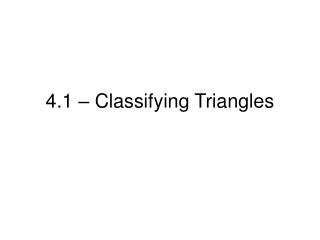Download Presentation4.1 – Classifying Triangles4.1 – Classifying Triangles - PowerPoint PPT Presentation

Download Presentation4.1 – Classifying Triangles
An Image/Link below is provided (as is) to download presentation

Download Policy: Content on the Website is provided to you AS IS for your information and personal use and may not be sold / licensed / shared on other websites without getting consent from its author. While downloading, if for some reason you are not able to download a presentation, the publisher may have deleted the file from their server.

- - - - - - - - - - - - - - - - - - - - - - - - - - - E N D - - - - - - - - - - - - - - - - - - - - - - - - - - -
Presentation Transcript

1. 4.1 – Classifying Triangles

2. Triangles • A polygon with three sides. • The corners are called vertices • A triangle with vertices A, B, and C is called “triangle ABC” or “

3. Classifying Triangles by Sides

4. Classifying Triangles by Angles

5. Example 1:Classify triangles by sides and angles a) b) c) 7 40° 15° 25 24 70° 70° 120° 45° • Solutions: • Scalene, Right • Isosceles, Acute • Scalene, Obtuse

6. Example 2:Classify triangles by sides and angles Now you try… a) b) c) 5 110° 3 5 5 4 5

7. Review: The distance formula To find the distance between two points in the coordinate plane…

8. Classify PQOby its sides. Then determine if the triangle is a right triangle. Use the distance formula to find the side lengths. STEP1 2 2 – – ( ( ) ) OP = + + 2 2 – – ( ( ) ) y x x y y y x x 2 1 2 2 2 1 1 1 2 2 ( – ( ) (– 1 ) ) 0 2 – 0 2.2 + = = 5 OQ = 2 2 ( – ( ) 6 ) 0 – 0 3 6.7 + = = 45 EXAMPLE 3 Classify a triangle in a coordinate plane SOLUTION

9. PQ = 2 2 ( – ) 6 (– 1 ) ) 3 – ( 2 7.1 + = = Check for right angles by checking the slopes. There is a right angle in the triangle if any of the slopes are perpendicular. STEP2 The slope ofOPis 2 – 0 3 – 0 1 . – 2. The slope ofOQis = = – 2 – 0 2 6 – 0 2 – ( ) + 2 – ( ) so OPOQand POQ is a right angle. y y x x 1 1 2 2 50 ANSWER Therefore, PQOis a right scalene triangle. EXAMPLE 3 Classify a triangle in a coordinate plane (continued)

10. Example 4:Classify a triangle in the coordinate plane Now you try… Classify ΔABC by its sides. Then determine if the triangle is a right triangle. The vertices are A(0,0), B(3,3) and C(-3,3). Step 1: Plot the points in the coordinate plane.

11. Example 4: (continued)Classify a triangle in the coordinate plane Step 2: Use the distance formula to find the side lengths: AB = BC = CA = Therefore, ΔABC is a ______________ triangle.

12. Example 4: (continued)Classify a triangle in the coordinate plane Step 3: Check for right angles by checking the slopes. The slope of = The slope of = The slope of = Therefore, ΔABC is a ______________ triangle.